# Solve the follwing rational inequality: 2 – 3 < 0 x + 1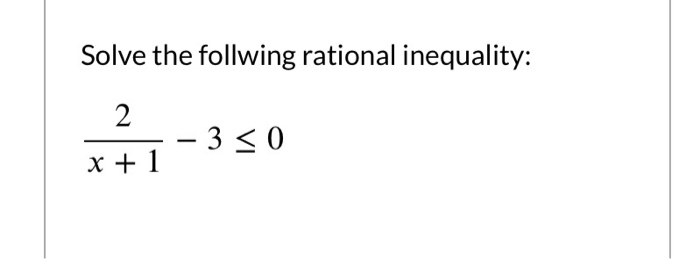Solve the follwing rational inequality: 2 – 3 < 0 x + 1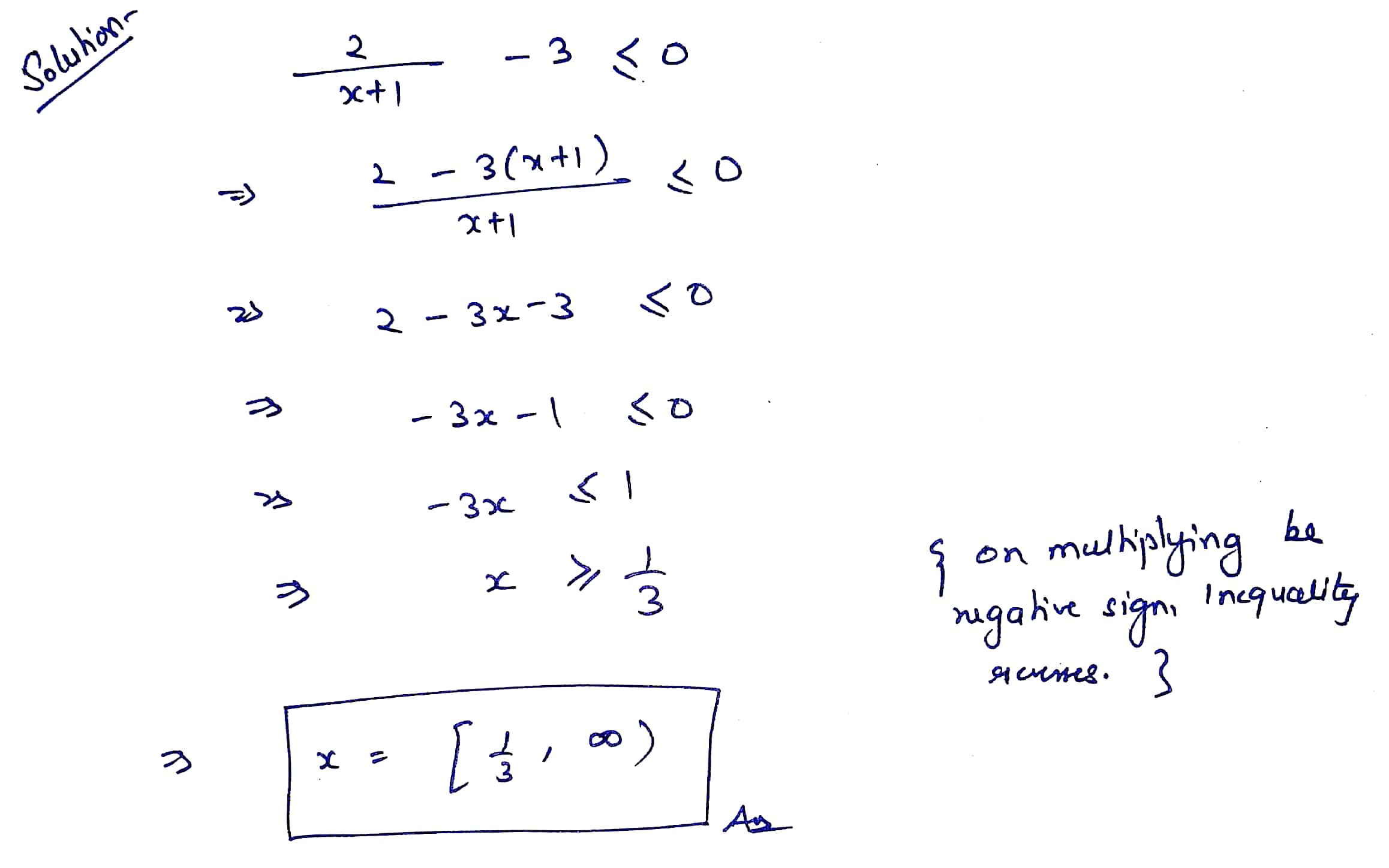Please give it a thumbs up if you like the answer. Comment if any problem in solution. :)

##### Add Answer of: Solve the follwing rational inequality: 2 – 3 < 0 x + 1
Similar Homework Help Questions
• ### Solve the inequality. 2x + 3 -1< 5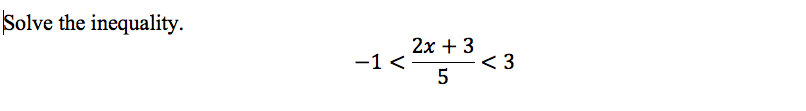Solve the inequality. 2x + 3 -1< 5

• ### PLEASE WRITE NEATLY!!! Solve the inequality 22 +2 - 2 22 - 5.0 + 6 <0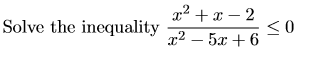PLEASE WRITE NEATLY!!! Solve the inequality 22 +2 - 2 22 - 5.0 + 6 <0

• ### Solve the inequality 22 +2 - 2 22 - 5.0 + 6 <0Solve the inequality 22 +2 - 2 22 - 5.0 + 6 <0

• ### Solve the inequality log2 (x2 – 3x + 4) <1. Give the answer as an interval.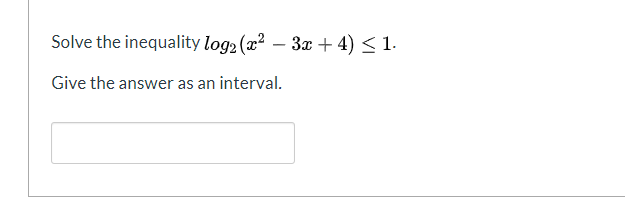Solve the inequality log2 (x2 – 3x + 4) <1. Give the answer as an interval.

• ### Solve the inequality f(x) <0, where f(x) = - x2(x + 4), by using the graph...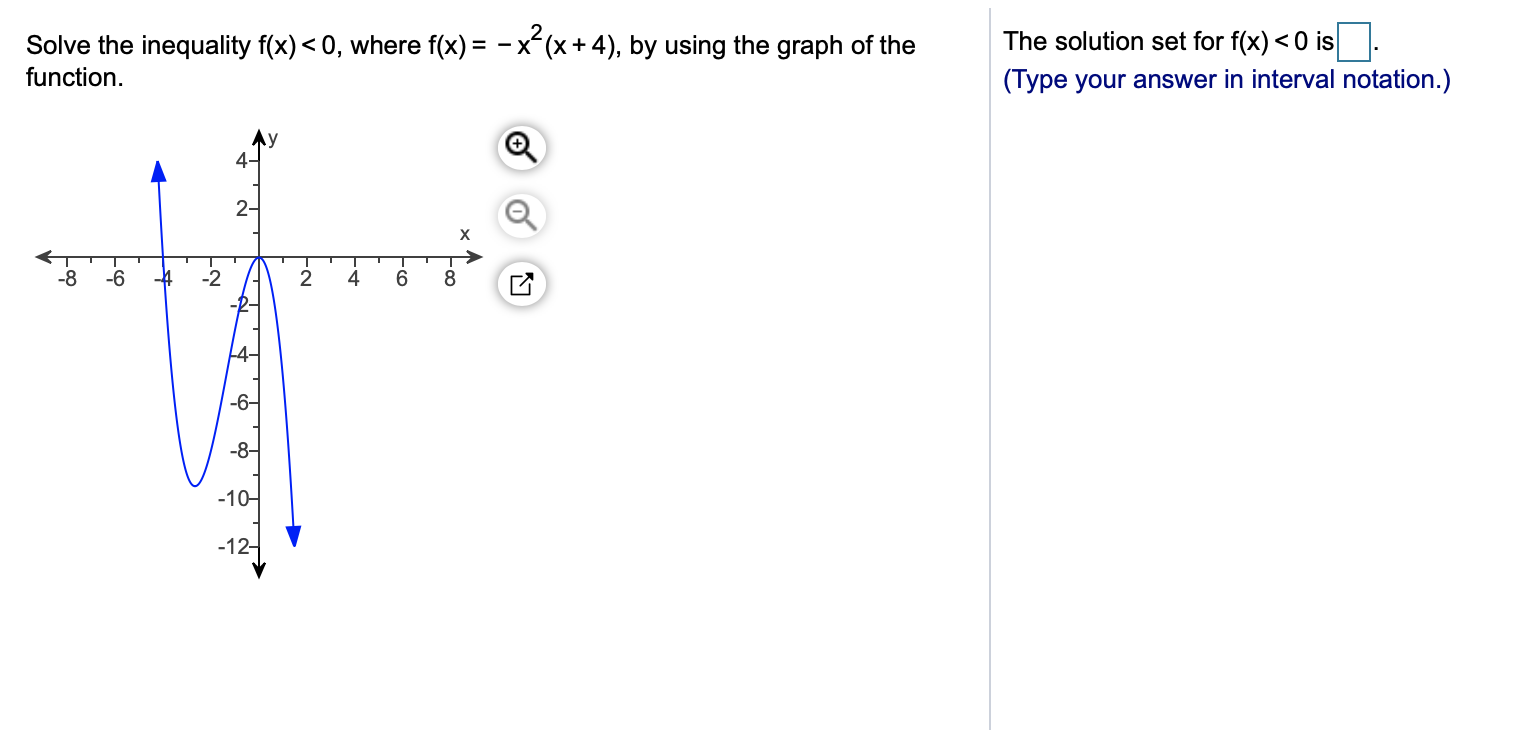Solve the inequality f(x) <0, where f(x) = - x2(x + 4), by using the graph of the The solution set for f(x) <0 is. (Type your answer in interval notation.) function. Ay 4- 2- х 500 -8 -6 -4 -2 2 4. 6 -8- -104 -12-

• ### 5. Which inequality is graphed on this number line ? -5 4 -3 -2 -1 0...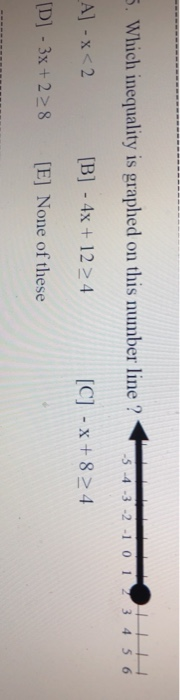5. Which inequality is graphed on this number line ? -5 4 -3 -2 -1 0 1 3 4 5 6 _A] -x<2 [B] - 4x + 12 4 [C] - X+824 [D] - 3x + 28 [E] None of these

• ### Find the fourier series و = (x) 1, 18, - 7<<0 0 << ;}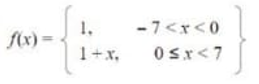Find the fourier series و = (x) 1, 18, - 7<<0 0 << ;}

• ### 10.( /4 points) Solve the equation for 0 if 0° 0 < 360°. V2 sec 0...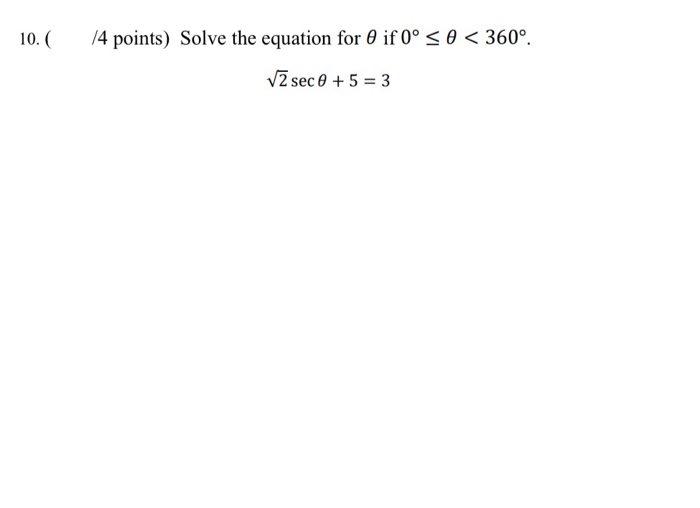10.( /4 points) Solve the equation for 0 if 0° 0 < 360°. V2 sec 0 + 5 = 3

• ### Solve the inequality. Express your answer using set notation or interval notation. Graph the solution set....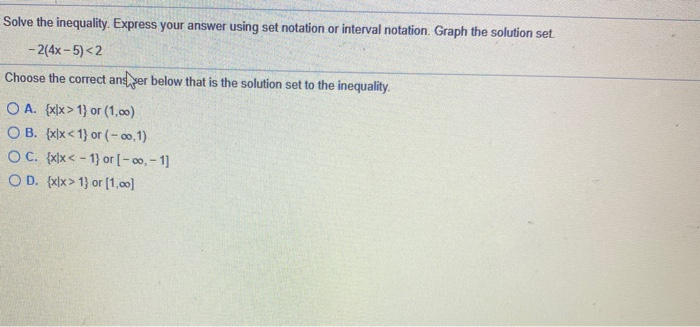Solve the inequality. Express your answer using set notation or interval notation. Graph the solution set. - 2(4x-5)<2 Choose the correct and per below that is the solution set to the inequality O A. {x[x> 1} or (1,00) OB. {x\x < 1) or (-0,1) O C. {x}x< - 1} or [-00,- 1] OD. {x[x> 1} or [1.00]

• ### 1. Solve the following DE: (50 pts) (1, if 0<x51 a) y+ y = f(x), y(0)...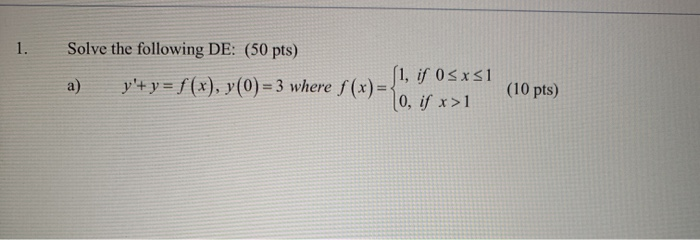1. Solve the following DE: (50 pts) (1, if 0<x51 a) y+ y = f(x), y(0) = 3 where f(x)= 0, if x>1 (10 pts)

Free Homework App